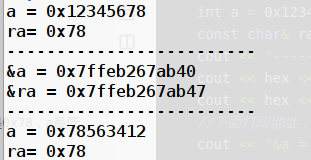# 引用类型和目标变量类型不一致，会发生什么？

C++中，引用类型和目标类型要保持一致，但是如果是常引用，当和目标类型不一致时，编译器是不会报错的

#include <iostream>
using namespace std;
int main(void){
int num = 10;
char& r1 = num;	//编译器会报错
const char& r2 = num;//编译器不会报错，可以正常编译
return 0;
}


#include <iostream>
using namespace std;
int main(void){
int a = 0x12345678;
const char& ra = a;
cout << "-------------------------" << endl;
cout << hex << showbase << a << endl;
cout << hex << showbase << (int)ra << endl;//结果是0x78，a最低位字节的数据
//下面打印地址，是不一样的
cout << "&a = " << &a << endl;
cout << "&ra = " << (void*)&ra << endl;
//重新给a赋值，再分别打印a和ra
cout << "-------------------------" << endl;
a = 0x78563412;
cout << hex << showbase << a << endl;
cout << hex << showbase << (int)ra << endl;
}©️2019 CSDN 皮肤主题: 游动-白 设计师: 上身试试